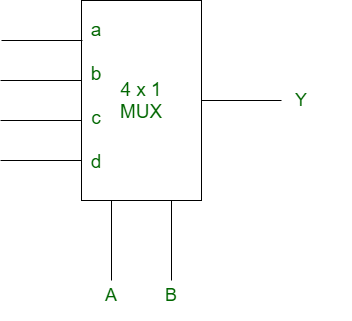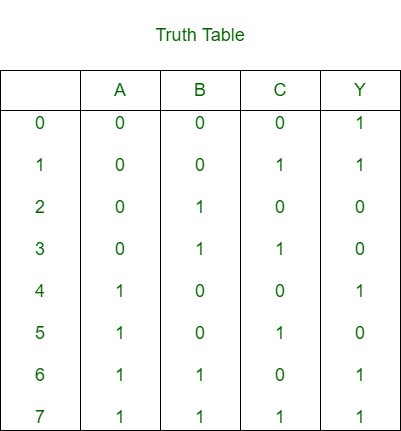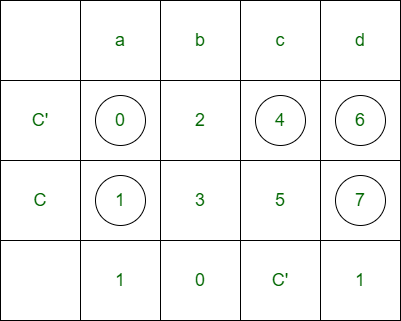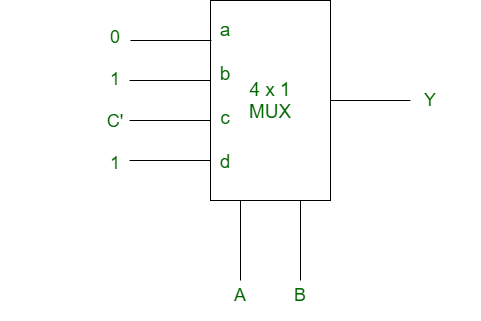Related Articles
Implementation of SOP function using multiplexer
• Difficulty Level : Easy
• Last Updated : 28 Apr, 2020

Given a SOP function and a multiplexer is also given. We will need to implement the given SOP function using the given MUX.

There are certain steps involved in it:
Step 1: Draw the truth table for the given number of variable function.
Step 2: Consider one variable as input and remaining variables as select lines.
Step 3: Form a matrix where input lines of MUX are columns and input variable and its compliment are rows.
Step 4: Find AND between both rows on the basis of the truth table.
Step 5: Hence whatever is found is considered as input of MUX.

We will illustrate it with an example:

Example:
Given SOP function f(A, B, C) = m(0, 1, 4, 6, 7) and MUX isFor 3 variable function, the truth table isLet A and B are the select lines and C be the input,Thus, for the implementation of given logical function, required is one 4×1 MUX and and inverter.Attention reader! Don’t stop learning now. Get hold of all the important CS Theory concepts for SDE interviews with the CS Theory Course at a student-friendly price and become industry ready.

My Personal Notes arrow_drop_up
Recommended Articles
Page :